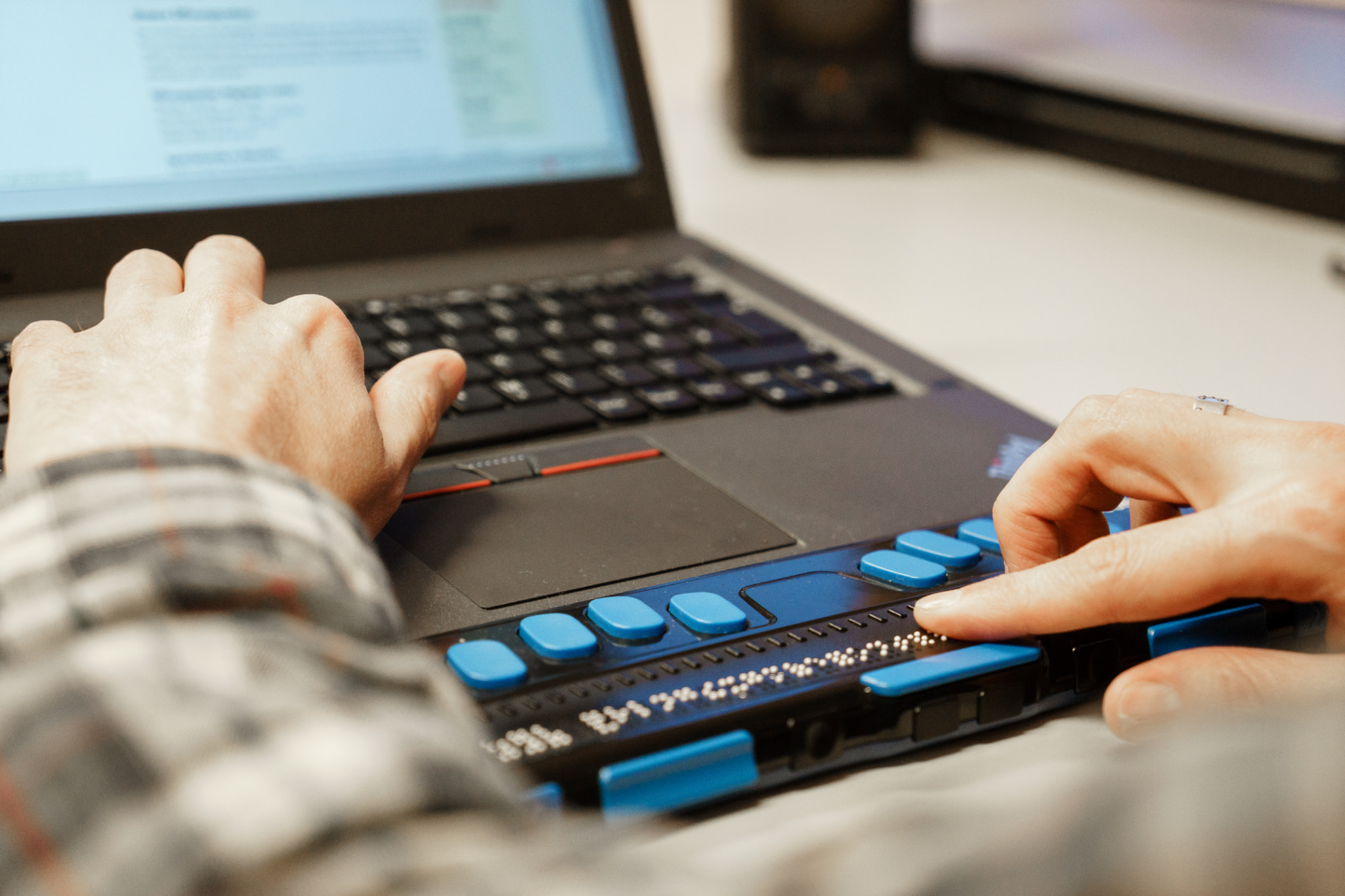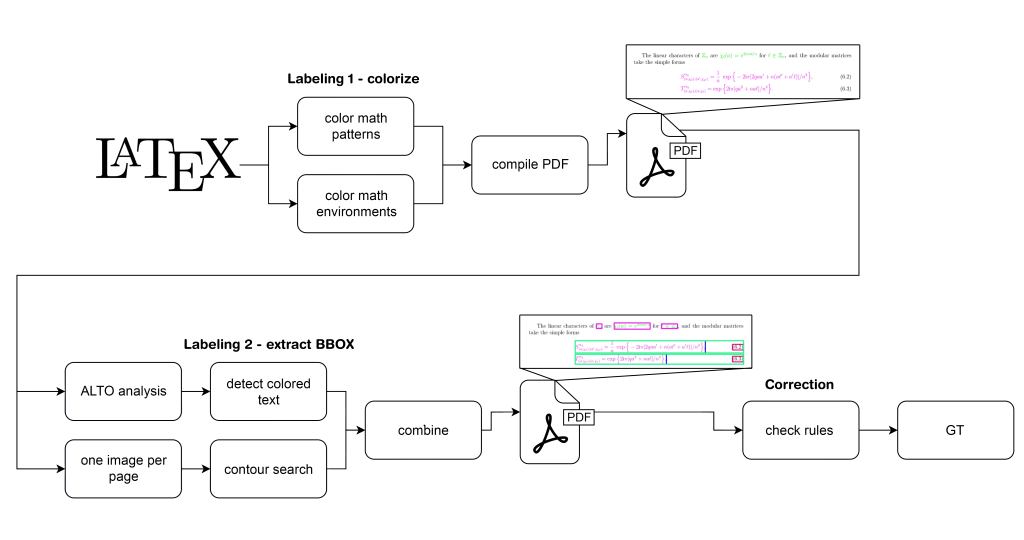# New database helps AI recognize mathematical formulae

Artificial intelligence (AI) is making headlines recently. AI tools that can create text or images (e.g., ChatGTP or Dall-E) are on the rise. Felix Schmitt-Koopmann asked himself how AI could automatically detect mathematical formulae in a text and ultimately help visually impaired people. He created the new database “FormulaNet” to take a step closer on the road to accessible scientific PDFs.

In his Ph.D., Felix takes on a big problem: PDFs are often not accessible and even if the author of a PDF wants to remedy this, it is not an easy task. A person with visual impairment uses assistive technologies, e.g., screen readers (= a tool that converts content into audio or braille), to navigate and read PDFs. The navigation is only possible if the author of the document adds extra information, like tags about the logical structure of the text and alternative text for images. In their study, Alireza Darvishy et. al. find that scientific PDFs are rarely tagged because the process is complicated and time-consuming. Consequently, people with visual impairments in the STEM field often do not have access to their content, especially the mathematical formulae. Felix applies AI for document analysis to automate the tagging process of PDFs – making it easier for the authors to create accessible papers.

#### Felix, how can you automatically tag a mathematical formula?

Tagging a mathematical formula in a PDF requires two steps. In a first step you start by detecting the position of the mathematical formula in the document. This object detection task is called – you might have guessed – mathematical formula detection. In a second step, the mathematical formula must be translated into an alternative text. This is an image2text task termed mathematical formula recognition.

#### And why is it so difficult to automate these two tasks?

Mathematical formula detection is challenging, because existing datasets are small compared to other object detection datasets and the differentiation between mathematical formula and text is not always clear. So, we had to precisely define what counts as a formula. We established two different formats: display formula (isolated from the text) and inline formula (incorporated in the text). The most important thing for the automatic detection is clearly defined rules so that the AI model detects the patterns we are looking for.

The second step, mathematical formulae recognition, is difficult for AI, as the style of a symbol can influence its meaning, and mathematical formulae are represented by a vast number of symbols. Especially challenging is the detection of 2D positions: Subscript and superscript (x0, x2). The most used dataset (im2latex-100k) uses LaTeX code as alternative text. LaTeX code adds the difficulty that different LaTeX codes can have the same outcome.Output Ground Truth (GT) = bounding boxes of the detected objects.

#### In this paper, you focused on the first step – the detection task. How does your new dataset help overcome the challenge of detecting formulae in a text?

AI models are only as good as the dataset. If we use a small dataset with few mathematical formulae, our model has a limited output. For our goal (automatic detection of mathematical formulae) we therefore had to establish a new high-quality and large-scale dataset. It is based on scientific papers from arXiv.org and contains 45k pages of physics papers. They contain many mathematical formulae – exactly what we needed. After creating the dataset, we established the task for an automatic detection. To achieve this, the LaTeX code of the papers was analysed: patterns that suggested a formula were highlighted. This is a common approach to extract objects in documents but we refined it to achieve more precise results for mathematical formulae.

#### How do you know that the results were more precise?

We know that because we conducted three experiments. The results confirmed that with our new dataset we not only have a good quantity of instances, but also a better annotation of formulae.

#### Who can use your new dataset and the automated labelling pipeline?

Both the paper and the new dataset are open access. Researchers can use our advanced fully automated labelling pipeline for constructing similar high-quality datasets of page object detection (POD) of nearly any size.

#### Your goal is to create “accessible scientific PDFs for all”. What’s next on your journey?

With the current paper we can detect where a mathematical formula is. The formula will then be cut out as an image. In a second step, I will train an image-to-text model, where the model predicts the alternative text for an image of a formula. These models will be integrated in PAVE (our tool for accessible PDFs), helping the authors of a PDF to faster and more easily tag it and consequently make the PDF accessible. I want to improve this process so that more scientists make their papers accessible. In the end, this helps visually impaired people to access scientific content.